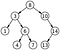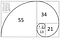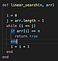# Breadth First Search

Breadth first search is a common technique to search a tree data structure. As in the name, this algorithm traverses the tree shallow before going deeper. A tree usually consists of nodes or vertices that hold a value as well as point to other values, which links these items together in a tree like structure.

Let’s look at some pseudocode for how to implement the algorithm, this uses key value pairs to keep track of visited nodes and a queue to keep track of the next nodes to visit:

1. Pick a node to start with and add it to the…

# Depth First Search and Simple Recursion

Today we’ll be talking about a technique to search through a tree called Depth First Search. One common implementation of a tree is a binary tree. A binary tree is a data structure consisting of nodes. Each node contains a value and a pointer to the next two nodes in the tree, right and left. A binary tree can be visualized as below:Binary Tree

There are many ways to search this data structure, and one is Depth First Search (DFS). DFS starts at a node, and searches through the tree one node at a time until it finds a node that…

# Implementing Quick Select in Ruby

In this blog, we’ll walk through how to write the Quick Select algorithm in Ruby. This technique is used to find the kth smallest element in an unsorted array, with an average runtime complexity of O(n). Quick Select uses a similar approach to the popular Quick Sort algorithm. It is a divide and conquer approach where the array to search is cut into smaller and smaller pieces until the desired result is found.

At first glance, finding the kth smallest element of an array is simple. Just sort the array and pick the element at the index [k - 1]…

# Implementing Quicksort in JavaScript

In this blog, we implement a Quicksort in JavaScript. Quicksort is an algorithm used to sort arrays with a divide and conquer technique. First, we select a pivot element of the array, in this case the middle element. Next, we swap elements that are greater than or less than the pivot and produce 2 sub-arrays. When successful, the sub-array on the right only contains elements that are greater than the pivot, and the one on the left only contains elements that are less than the pivot. After this step, we know that the pivot element has found its’ final position…

# Recursion, Fibonacci, and Speed with Ruby

The Fibonacci sequence is a sequence of numbers where the next number in the sequence is the sum of the previous 2 numbers. It is typically illustrated like this:Fibonacci Sequence

In this blog, I’ll be dipping my toes into the basics of how recursion works. I’ll implement two different solutions in Ruby to find the element of the Fibonacci sequence specified. The first solution is more standard coding practice while the second uses a more elegant, recursive solution to do the job.

The first solution takes in a number n, and creates a Fibonacci sequence from the beginning all the way…

# Linked Lists vs. Arrays

As I try to wrap my brain around all of these different data structures that exist in the world of programming, today I’m looking into linked lists and how they differ from arrays. Each structure is a way to store groups of data and provide different advantages and disadvantages when working with the structure.

# Binary vs. Linear Search and Ruby’s .find Method

I’m currently learning the Ruby programming language, and I was curious what kind of search is used in Ruby’s .find method for arrays. After a bit of searching around the docs, then figured why not find out for myself. I was interested to see what method of searching .find uses, and whether it was the fastest method to search through arrays or not.

To find this out, I wrote Ruby methods for linear search and binary search, implemented them, and tested their benchmarks with different sized arrays.

Here is how I implemented linear search:Linear Search in Ruby

Here is how I implemented binary…## Matt Sewell

Software Engineer